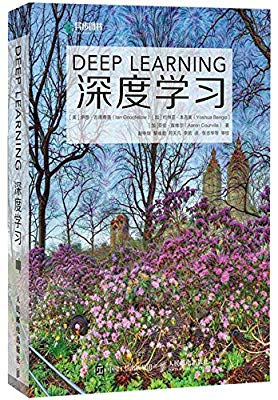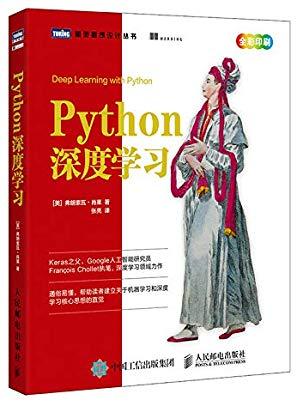# 京山游侠

## 前言##

JetBrains 大家都知道啦，JetBrains 全家桶是广大程序员的福利啦。做科学计算，使用 PyCharm 的 Community 版就足够了，没必要下载 Professional 版啦，我更加是不鼓励大家用破解版的啦。

## 环境安装##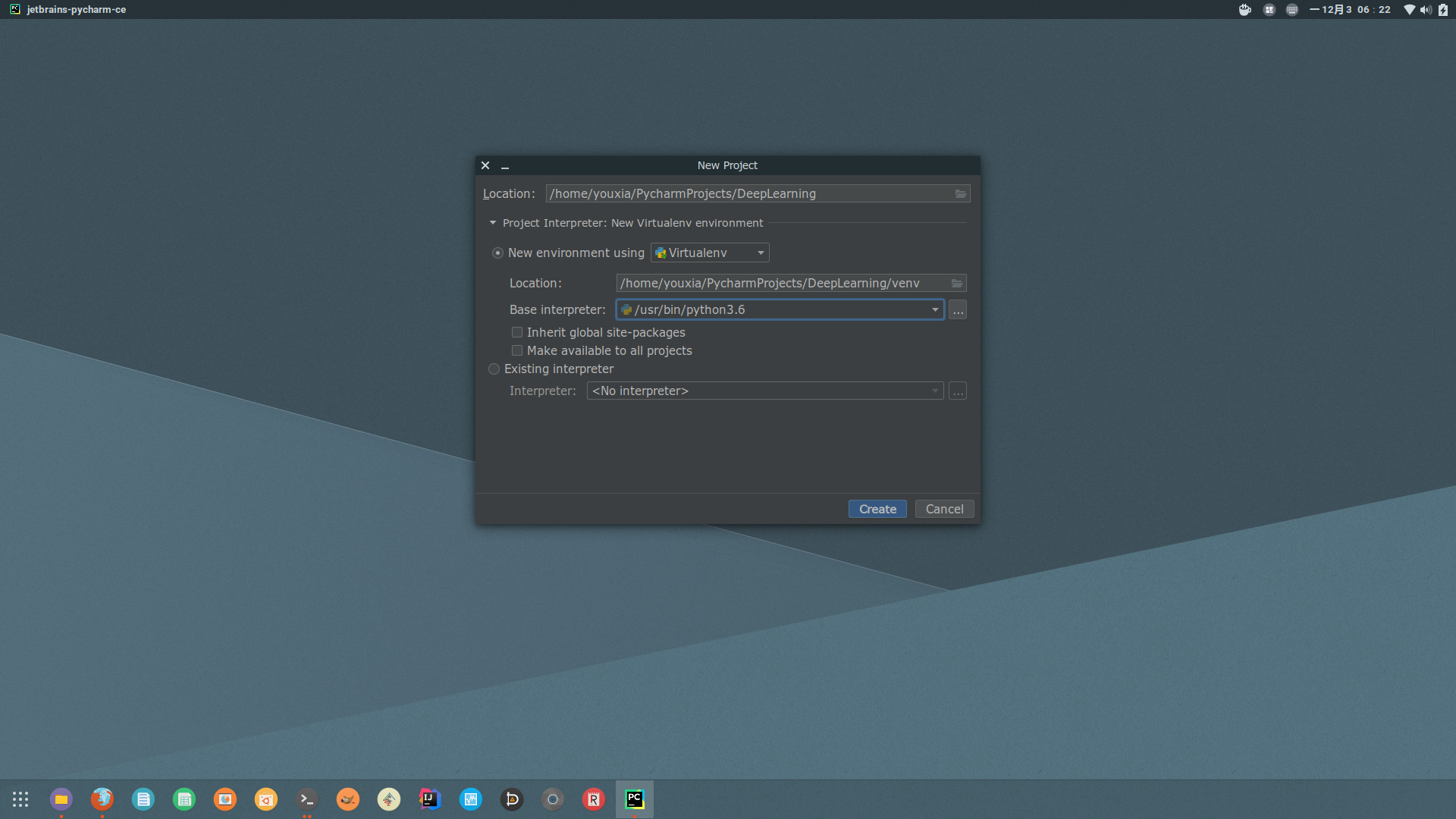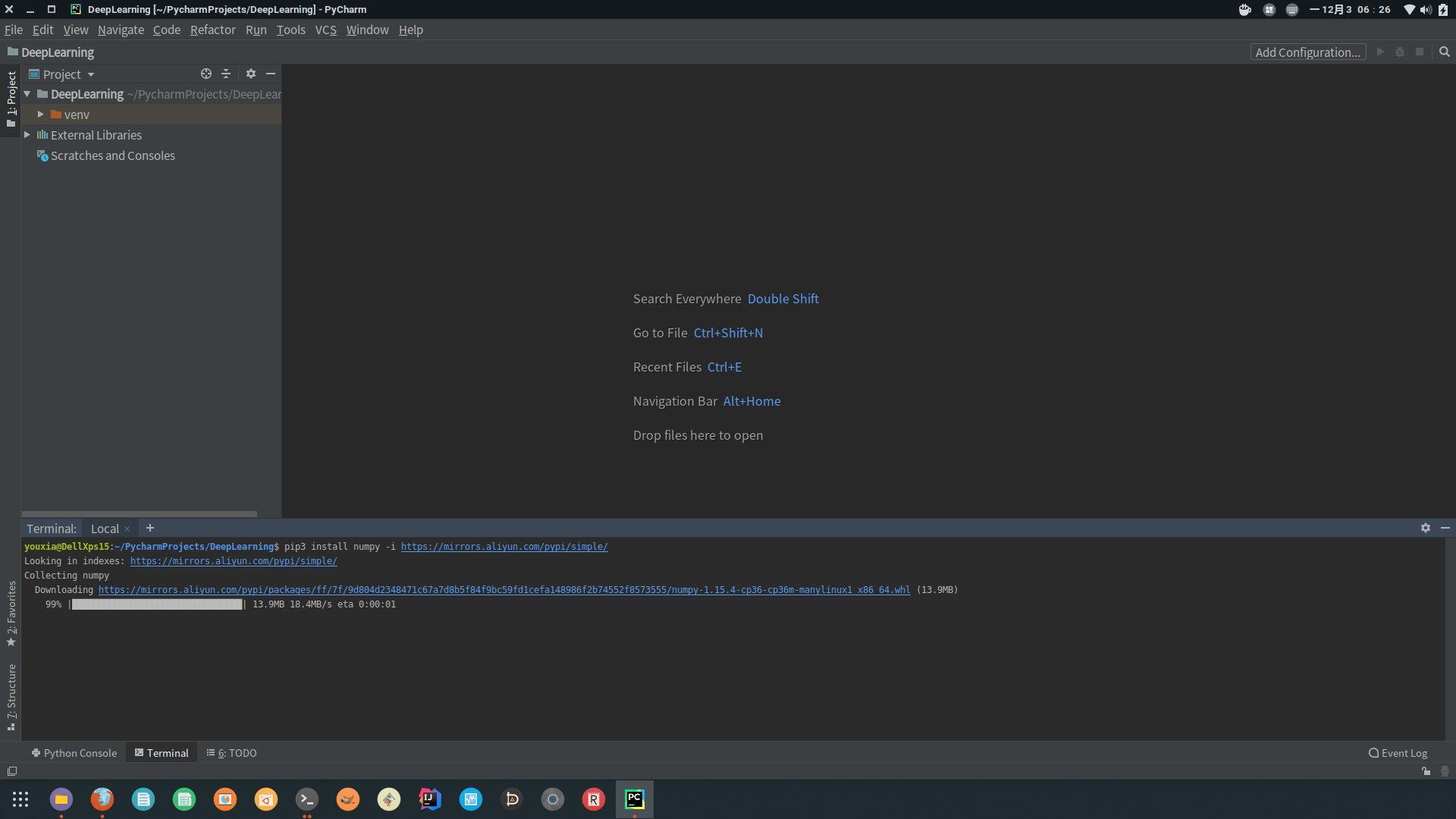pip3 install numpy -i https://mirrors.aliyun.com/pypi/simple/
pip3 install tensorflow -i https://mirrors.aliyun.com/pypi/simple/
pip3 install keras -i https://mirrors.aliyun.com/pypi/simple/
pip3 install matplotlib -i https://mirrors.aliyun.com/pypi/simple/
pip3 install Pillow -i https://mirrors.aliyun.com/pypi/simple/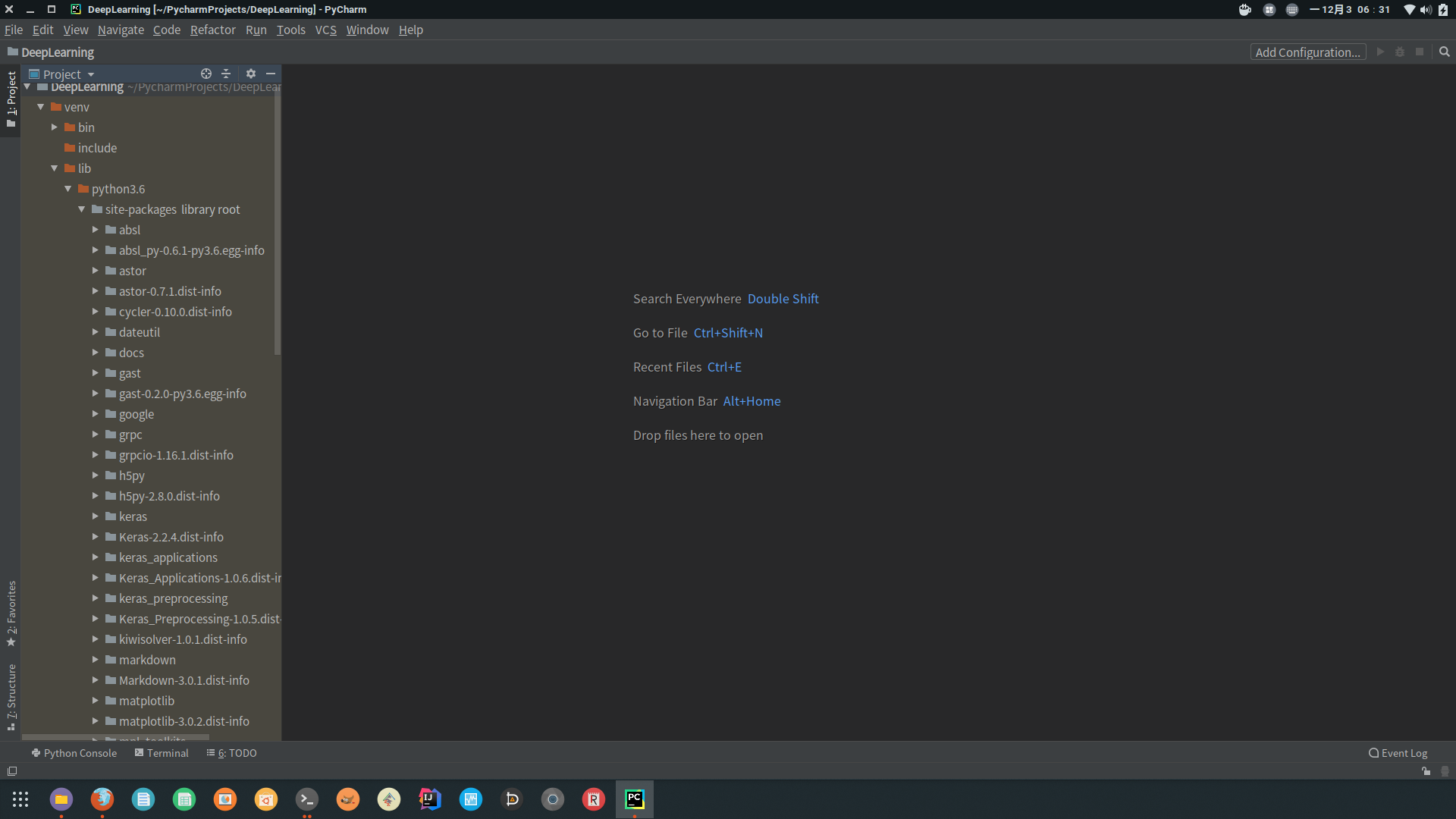PyCharm 最大的优势当然是它的自动代码提示了。不管是写一个.py文件，还是直接使用 PyCharm 中的 Python Console，就是有非常好的代码提示的。Python Console 还有一个非常棒的功能，就是可以观察每一个变量的值，真的是太方便了。如下图：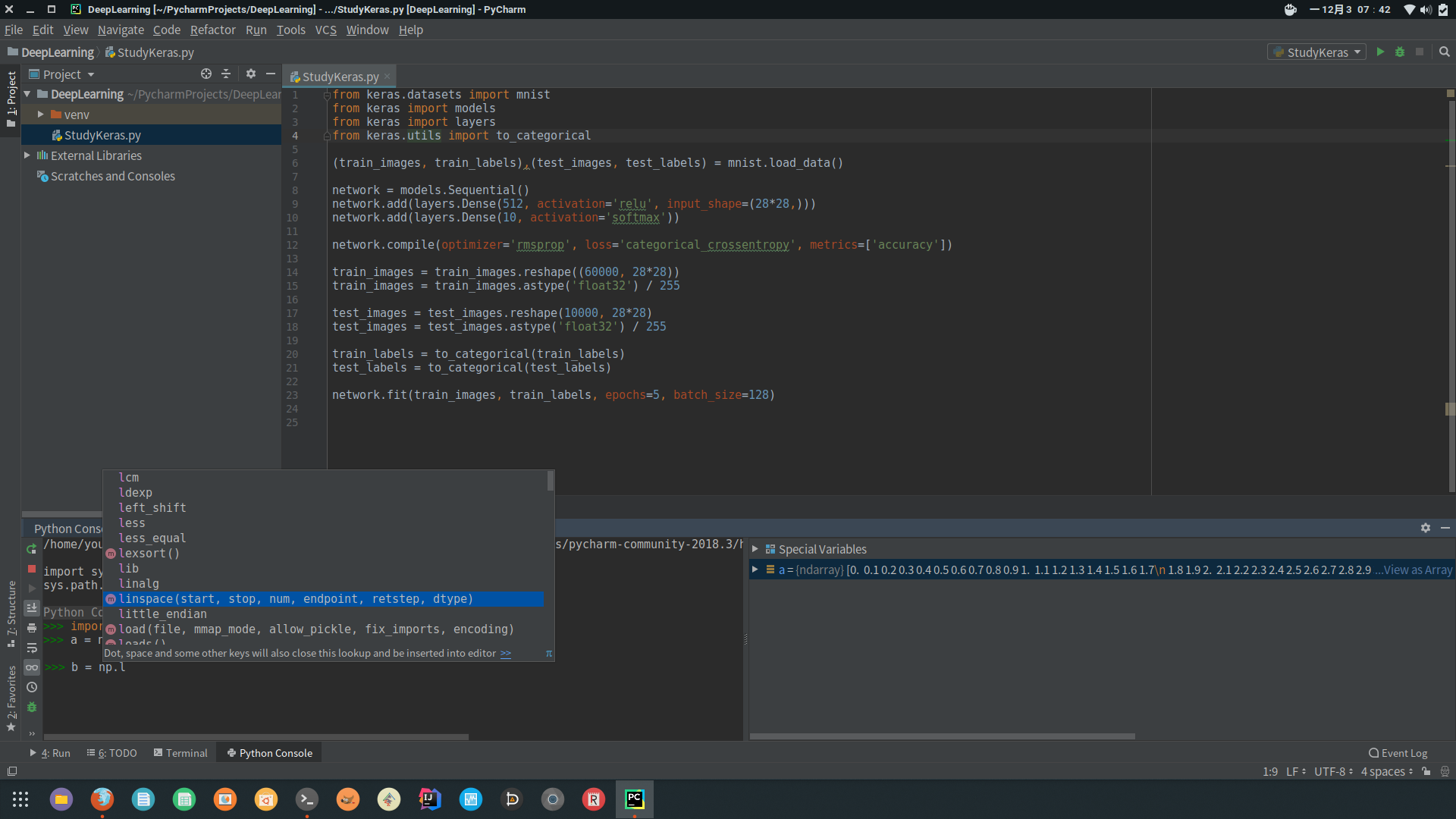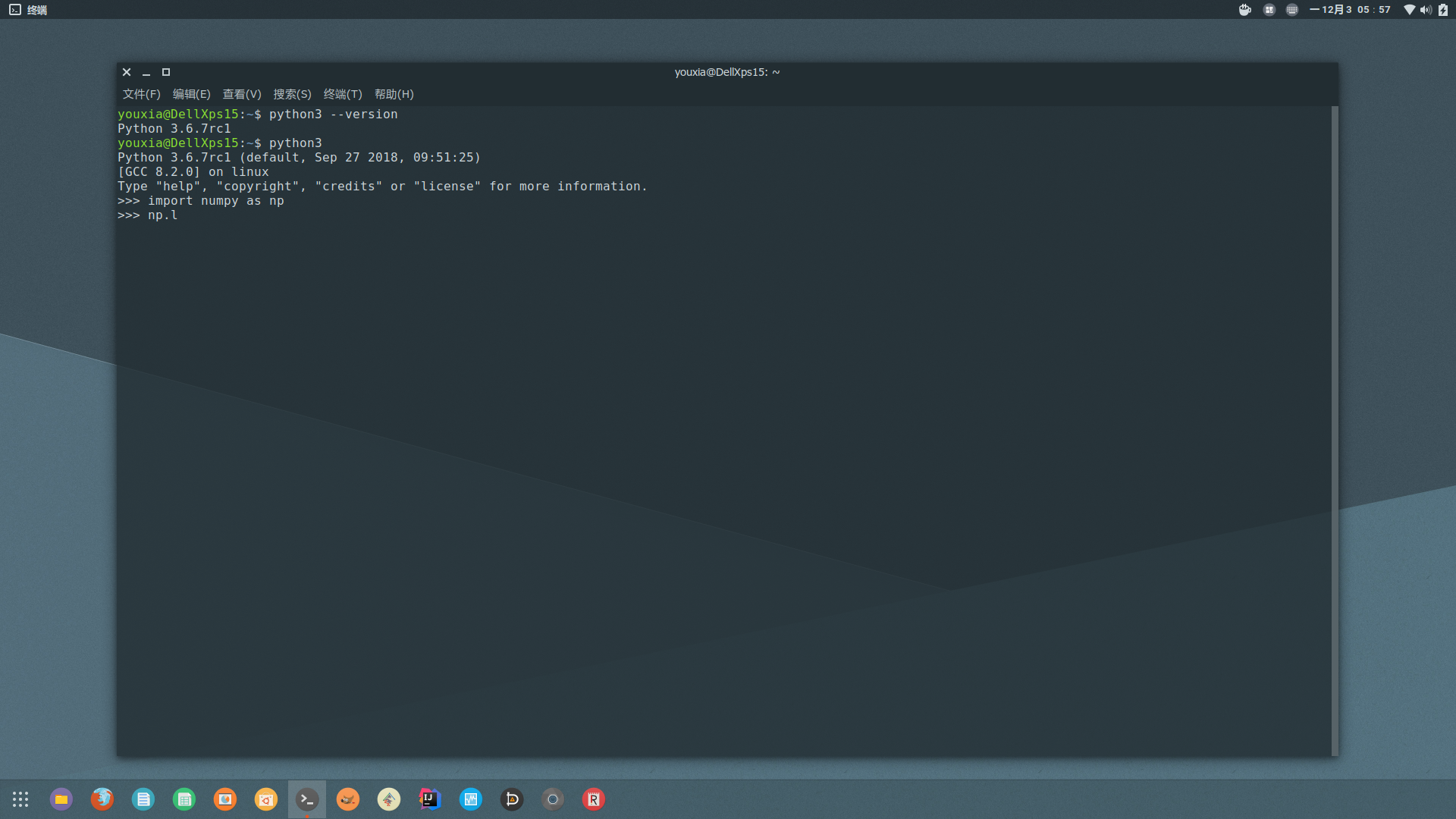## 最简单的深度学习示例##

from keras.datasets import mnist
from keras import models
from keras import layers
from keras.utils import to_categorical

network = models.Sequential()

network.compile(optimizer='rmsprop', loss='categorical_crossentropy', metrics=['accuracy'])

train_images = train_images.reshape((60000, 28*28))
train_images = train_images.astype('float32') / 255

test_images = test_images.reshape(10000, 28*28)
test_images = test_images.astype('float32') / 255

train_labels = to_categorical(train_labels)
test_labels = to_categorical(test_labels)

network.fit(train_images, train_labels, epochs=5, batch_size=128)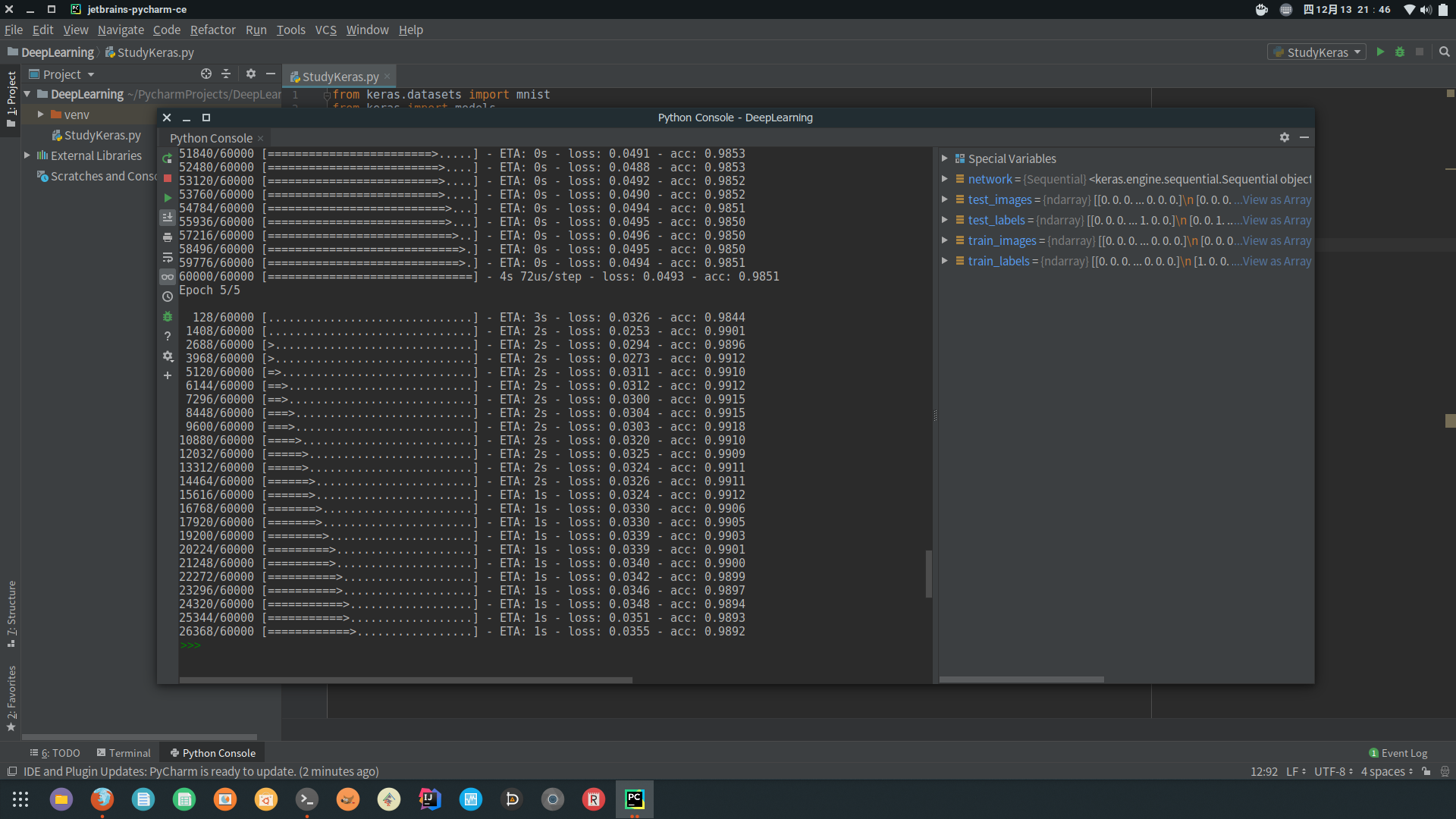from PIL import Image
image = Image.open('/home/youxia/test.png').resize((28,28)).convert('L')


import numpy as np
im = np.array(image)
im = 255 - im
im = im.astype('float32') / 255.0


import matplotlib.pyplot as plt
plt.imshow(im, cmap=plt.cm.binary)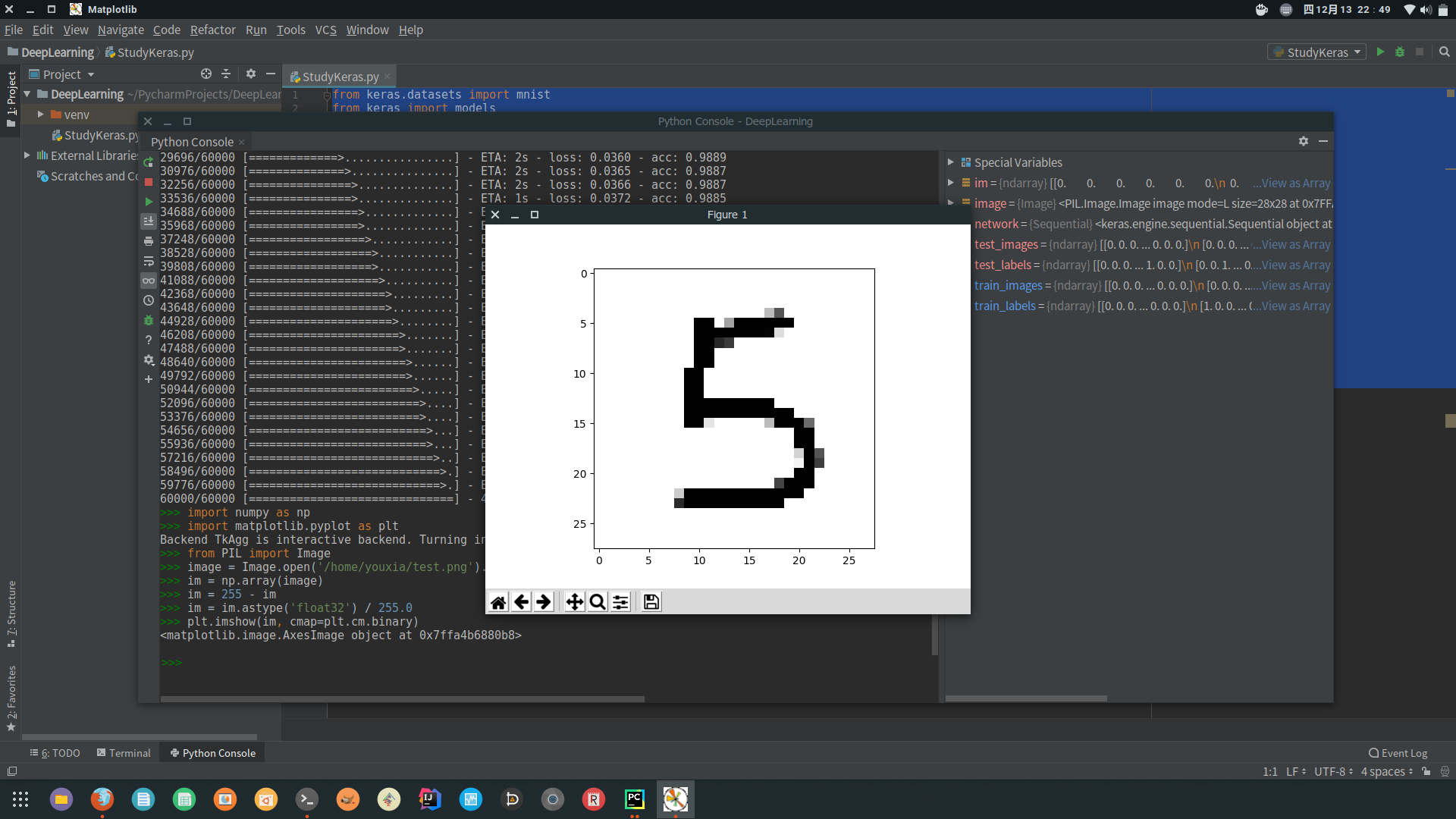network.predict(im.reshape(1,784))


array([[2.19245143e-11, 7.56166017e-13, 2.06190620e-10, 3.12406366e-04,
1.12510295e-13, 9.99686599e-01, 8.85503199e-11, 2.70172443e-11,
2.85677032e-07, 7.55779013e-07]], dtype=float32)


np.argmax(network.predict(im.reshape(1,784)))


5


## 从密集连接神经网络到卷积神经网络##

from keras import layers
from keras import models
from keras.datasets import mnist
from keras.utils import to_categorical

model = models.Sequential()
model.add(layers.Conv2D(32, (3, 3), activation='relu', input_shape=(28, 28, 1)))

model.compile(optimizer='rmsprop', loss='categorical_crossentropy', metrics=['accuracy'])

(train_images, train_labels), (test_images, test_labels) = mnist.load_data()
train_images = train_images.reshape((60000, 28, 28, 1))
train_images = train_images.astype('float32') / 255
test_images = test_images.reshape(10000, 28, 28, 1)
test_images = test_images.astype('float32') / 255

train_labels = to_categorical(train_labels)
test_labels = to_categorical(test_labels)

model.fit(train_images, train_labels, epochs=5, batch_size=64)


import numpy as np
from PIL import Image

im = np.array(Image.open('/home/youxia/test.png').resize((28,28)).convert('L'))
im = 255 - im
im = im.astype('float32') / 255.0

print(np.argmax(model.predict(im.reshape(1, 28, 28, 1))))


## 关于深度学习的理论和实战##